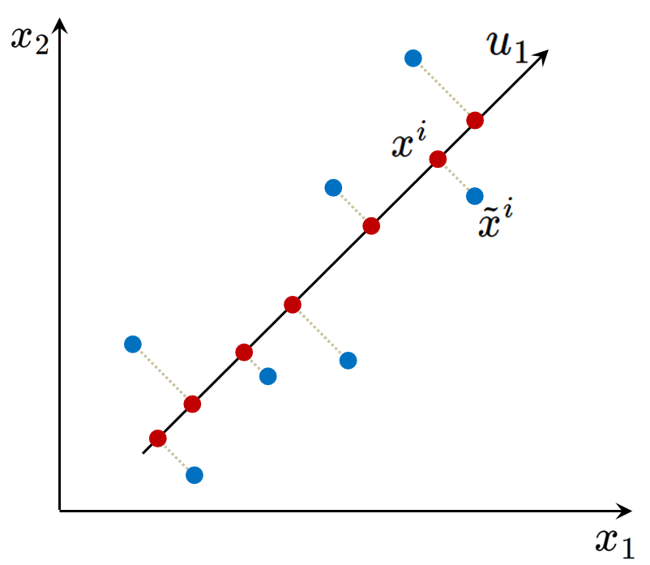Unsupervised Learning: Dimension Reduction

By Prof. Seungchul Lee
http://iai.postech.ac.kr/
Industrial AI Lab at POSTECH

# 1. Principal Component Analysis (PCA)¶

Motivation: Can we describe high-dimensional data in a "simpler" way?

$\quad \rightarrow$ Dimension reduction without losing too much information
$\quad \rightarrow$ Find a low-dimensional, yet useful representation of the data

• Why dimensionality reduction?
• insights into the low-dimensinal structures in the data (visualization)
• Fewer dimensions ⇒ Less chances of overfitting ⇒ Better generalization
• Speeding up learning algorithms
• Most algorithms scale badly with increasing data dimensionality
• Less storage requirements (data compression)
• Note: Dimensionality Reduction is different from Feature Selection
• .. although the goals are kind of the same
• Dimensionality reduction is more like “Feature Extraction
• Constructing a small set of new features from the original features
• How?
idea: highly correlated data contains redundant features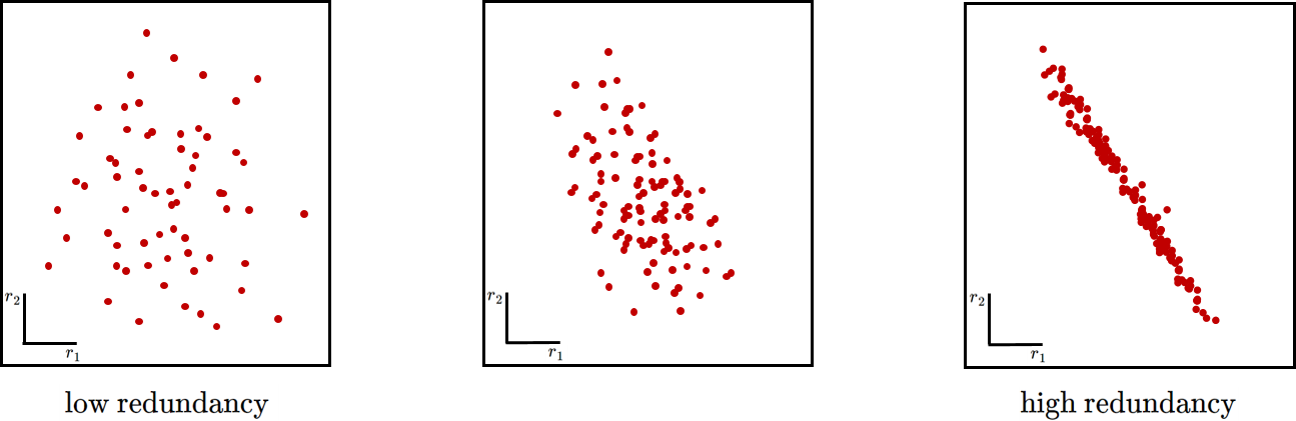• Each example $x$ has 2 features $\{x_1,x_2\}$

• Consider ignoring the feature $x_2$ for each example

• Each 2-dimensional example $x$ now becomes 1-dimensional $x = \{x_1\}$

• Are we losing much information by throwing away $x_2$ ?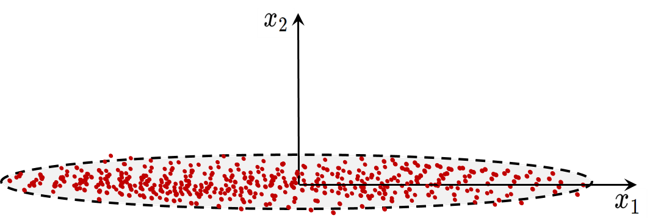• Each example $x$ has 2 features $\{x_1,x_2\}$

• Consider ignoring the feature $x_2$ for each example

• Each 2-dimensional example $x$ now becomes 1-dimensional $x = \{x_1\}$

• Are we losing much information by throwing away $x_2$ ?

• Yes, the data has substantial variance along both features (i.e. both axes)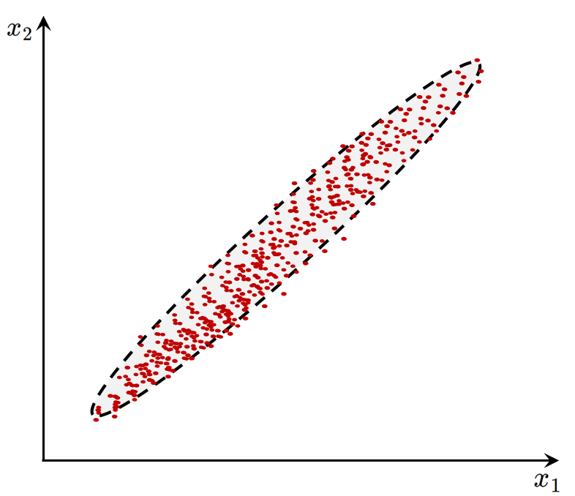• Now consider a change of axes

• Each example $x$ has 2 features $\{u_1,u_2\}$

• Consider ignoring the feature $u_2$ for each example

• Each 2-dimensional example $x$ now becomes 1-dimensional $x = \{u_1\}$

• Are we losing much information by throwing away $u_2$ ?

• No. Most of the data spread is along $u_1$ (very little variance along $u_2$)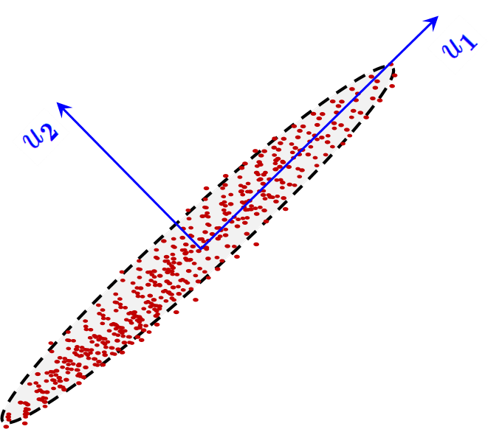• Data $\rightarrow$ projection onto unit vector $\hat{u}_1$
• PCA is used when we want projections capturing maximum variance directions
• Principal Components (PC): directions of maximum variability in the data
• Roughly speaking, PCA does a change of axes that can represent the data in a succinct manner